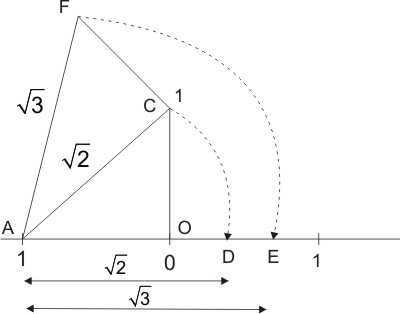# Representing rational and irrational numbers on number line

Representing Natural numbers, Whole numbers, Integers on the number line is easy.  The interesting part is with Rational numbers (Q) which are of the form $$\dfrac{p}{q}$$ where $$q \ne 0$$.  We know that all rational numbers are either perfect decimals ($$\because$$ 0.25, 1.46, 5.3456 etc) or recurring decimals. ($$\because$$ $$1.23\overline {45}$$, $$0.\overline 3 = \dfrac{1}{3}$$ etc).  These two types of numbers can be converted to $$\dfrac{p}{q}$$ format.  We can represent the perfect decimals like 1/4, or 1/8 easily on the number line.  Then what about $$\dfrac{1}{3}$$?### Representing 1/3 on the real number line:

We know that $$\dfrac{1}{3}$$ is a recurring decimal and goes on infinitely.  My question is how do we measure the length of this number?
To solve this interesting problem I took the help of geometry.  Let F, D, B are the points on y-axis an d "C" is of one unit length. When you connect B and C, It forms $$\Delta ABC$$.  Now draw a line parallel to x - axis from D to E and F to G.  As per mean proportionality theorem, we can easily prove that, $$BE:EG:GC = 1:1:1$$Now draw perpendiculars from E and G to x-axis.  As GJ, EH are parallel to y axis, they divide AC in the same ratio of $$CG,~GE,~EB$$.
Therefore, $$CJ:JH:HA = 1:1:1$$  and each of these parts equal to 1/3

### Representing $$\sqrt 2$$ on the real number line:

Another interesting question is how do we represent $$\sqrt 2$$ on the number line. The decimal numbers which cannot be written in the format of $$\dfrac{p}{q}$$ are irrational numbers.  They are non repeating and non recurring decimals.  For example, $$\sqrt 2$$ = 1.414213562373095048801688724209698078569671875376948073176...
You can see there is no pattern in the above decimal representation. How can we represent this number on the number line? We can done this using Pythagorean theorem.From the diagram, OC = 1 unit, OA = 1 unit. Now
$$AC$$ = $$\sqrt {O{A^2} + O{C^2}}$$  = $$\sqrt {{1^2} + {1^2}} = \sqrt 2$$
Now AD = $$\sqrt 2$$

### Concept of irrationality:Let us take two sets $$A$$ and $$B$$ where all the numbers lie to the left of $$2$$ on the number line is set $$A$$ and all the numbers lie to the right of 2 is set $$B$$.  Clearly each element of set $$B$$  is greater than each element in set$$A$$.
If $$2$$ belongs to set$$A$$, it is the largest element in set A. If $$2$$ belongs to set$$B$$, it is the smallest element in set$$B$$.  You cannot find a smaller element than $$2$$ in set$$B$$.
Therefore, Rational number is defined as this number will be either largest of SetA or Smallest of SetB

When you look at the number like $$\sqrt 2$$, the decimal representation goes on forever without any recurrence of numbers.  Then how do we determine the exact length of $$\sqrt 2$$ on number line.  Representation of irrational numbers on the number line is possible by using "Dedekind's cut"Let $$x = \sqrt 2 \Rightarrow {x^2} = 2$$
Square of some number is 2. Let there exist $${a^2},~{b^2}$$ such that $${a^2} < 2 < {b^2}$$ where $${a^2}$$ belongs to set to the left of $$\sqrt 2$$ and $${b^2}$$ belongs to the set right of $$\sqrt 2$$.
Interestingly, $$\sqrt 2$$ is not the largest of set$$A$$, nor is the smallest of set$$B$$.  Because we can find some $${k^2}$$ which is greater than $${a^2}$$ but less than $$\sqrt 2$$ (or) some $${m^2}$$ which is greater than $$\sqrt 2$$ but less than $${b^2}$$).
$${a^2} < {k^2} < 2 < {m^2} < {b^2}$$
So this indicates the irrational nature of  $$\sqrt 2$$.  That means, we can only "approach" the value of $$\sqrt 2$$.
Irrational number is defined as this number will be neither largest of SetA nor Smallest of SetB

Finally:
Without irrational  numbers the number line is not complete. In other words, there exist some gaps between numbers.  Irrational numbers fill these gaps and make number line continuous. The set of irrational numbers is infinite and uncountable.